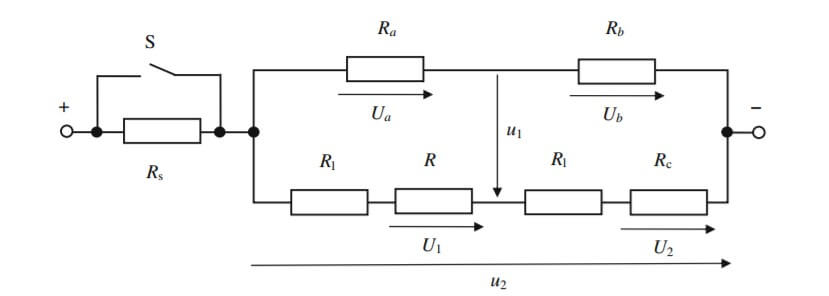# DIY: Transient Hot Strip

## Theory:

Consider a strip of infinite length, width $$2a$$, and no thickness. Let the strip generate heat flow, $$\phi$$, deposited evenly into an infinite slab of properties, $$\lambda$$, $$\kappa$$.  placed on top of it. If the strip lies divided by the y-axis, then the temperature distribution at the surface will follow:

\begin{aligned}T(x,y,0,t) =& \frac{\phi\tau^{\frac{1}{2}}}{\lambda\pi^{\frac{1}{2}}}\Bigg[\text{erf}\Bigg(\frac{a+x}{2a\tau^{\frac{1}{2}}}\Bigg) \\ &+\text{erf}\Bigg(\frac{a{-}x}{2a\tau^{\frac{1}{2}}}\Bigg){-}\frac{a+x}{2a(\pi\tau)^{\frac{1}{2}}}\text{Ei}\Bigg({-}\frac{(a+x)^{2}}{4a^{2}\tau} \Bigg){-}\frac{a{-}x}{2a(\pi\tau)^{\frac{1}{2}}}\text{Ei}\Bigg({-}\frac{(a{-}x)^{2}}{4a^{2}\tau}\Bigg)\Bigg]\end{aligned}

Where $$\tau=\kappa{t}/a^{2}$$, and $$\text{Ei}$$ is the exponential integral, $$\text{Ei}({-}x)=\int_x^\infty\frac{e^{-t}}{t}dt$$. For the very center of the strip, the surface temperature of the material follows:

$T(0,0,0,t)=\frac{2\phi\tau^{1/2}}{\lambda\pi^{1/2}}\left[erf\frac{a}{2a{\tau{}}^{\frac{1}{2}}}-\frac{a}{2a{(\pi{}\tau{})}^{1/2}}\text{Ei}{\left(-\frac{a^2}{4a^2\tau{}}\right)}\right]$

Reintroducing $$t$$, and bringing in the thermal effusivity,$$e=\lambda/\sqrt{\kappa}$$ , the temperature of the actual strip can be expressed by the first term in the previous equations

$T_{s}(t)=\frac{2\phi\sqrt{t}}{e\sqrt{\pi}}$

As with many transient methods, this model will be used to characterize the change in resistance of a heat source. When current is run through the strip, it will produce heat, and the temperature of the strip will increase. If the relationship between resistance and temperature is known, then the temperature can be known. The resistance will be the sum of the original resistance, and the new component, or

$R(t)={R}_{0}{+}a\Delta{T}(t)$

$a=\frac{R_{0}}{R}\frac{dR}{dT}$

This is neglecting contact resistance. With contact resistance, the electrical resistance will be

$R(t)=R_{0}{=}a\Delta{T}_{i}{+}a\Delta{T}(t)$

Where $$a\Delta{T}_{i}$$ represents the contact resistance. After some small characteristic time, $$t_{p}$$, this value will be approximately constant. The resistance then follows a linear equation with respect to the square root of time, or

$R(t)=A+m\sqrt{t}$

$m=\frac{2a\phi}{e\sqrt{\pi}}$

The thermal effusivity is then related to the inverse of the slope. The offset, $$A$$, is not linear in regions of low $$t$$, and so trying to fit a straight line to the first portion of the experiment is of no use.

The resistance of the source element has historically been measured with a Wheatstone bridge, as shown below. The resistance will depend on the total voltage from input to output, $$u_{2}$$, the voltage difference across the bridge, $$u_{1}$$, and the resistances of the remaining elements. Mathematically,

$R=(R_{c}+R_{l})\Big[\frac{U_{a}+u_{1}}{U_{b}{-}u_{1}}\Big]{-}R_{l}$

$U_{a}=u_{2}\frac{R_{a}}{R_{a}+R_{b}},U_{b}=u_{2}{-}U{a}$

The only value that should depend on time is $$u_{1}$$. To keep the apparatus as close to the mathematical model as possible, resistances should be chosen carefully; $$R_{a}=R_{b},R_{c}=R(0)$$. The initial resistance of the strip can be found by inserting $$R_{s}>>1$$ into the circuit, as shown below. Choosing these resistances ensures that the power coming out of the strip is as constant as possible.

More considerations must be made before constructing an apparatus. The model has two effective dimensions of heat flow, but only one has been considered in the solutions above. This is because only one dimension is needed for values at the surface. The perpendicular direction, however, must still be considered in practical applications. The penetration depth, $$p$$, of the heat wave depends on diffusivity. For a material of unknown diffusivity, choosing as thick a material as possible is best, so that the heat cannot reach the other surface.

The initial model was of an infinite strip heating an infinite slab. To achieve this in practical terms, choose the dimensions of the material such that the material has width much greater than that of the strip, and length much greater than the width of the strip again. As with choosing the thickness of the material, this keeps the heat well away from the relevant edges, and so the material is effectively infinite.

For a material of unknown diffusivity, even a thick one, keeping the test times as short as possible while still getting enough useful data is best. This means that experiment should be long enough for the resistance to become linear, but short enough that the heat does not significantly penetrate the material. Regions of measurement should begin somewhere above 0.5s and end somewhere below 2s.## Materials

• Nickel strip, length ~ five times the width
• Styrofoam pad larger than the strip
• Weight
• Resistors:
• 2 x Ra
• 2 x R1
• 1 x Rc (~R)
• 1 x Rs
• Arduino board
• Electrical clamps
• Material to test (low conductivity recommended)
• Insulating sheet (optional)

## Apparatus

To assemble the apparatus, first clip one electrical clamp onto each end of the nickel strip. Place the nickel strip onto the Styrofoam pad. Place the material on top of the nickel strip, and the weight on top of that. Assemble the circuit shown in the diagram, with the material and strip taking the place of the resistor R.

Electrically conductive materials are also good thermal conductors. This is why a low conductivity material is recommended. If the material is of high conductivity, it is strongly recommended that you place a thin, thermally insulating sheet between the strip and the material.

Rc can be found using Rs and a temporary Rc as described above, or the electrical resistivity of the nickel strip can be estimated by using its dimension.